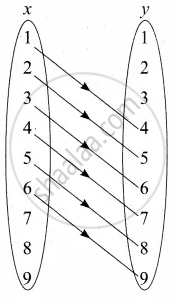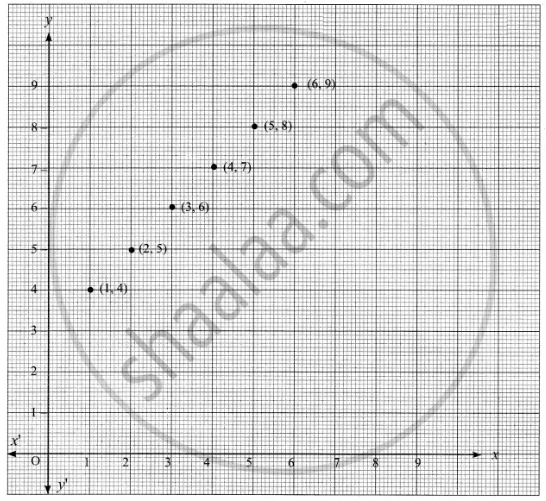Tamil Nadu Board of Secondary EducationSSLC (English Medium) Class 10th

# Represent the given relation by (a) an arrow diagram(b) a graph and (c) a set in roster form, wherever possible {(x, y) | y = x + 3, x, y are natural numbers < 10} - Mathematics

Diagram
Graph
Sum

Represent the given relation by
(a) an arrow diagram
(b) a graph and
(c) a set in roster form, wherever possible

{(x, y) | y = x + 3, x, y are natural numbers < 10}

#### Solution

x = {1, 2, 3, 4, 5, 6, 7, 8, 9}

y = {1, 2, 3, 4, 5, 6, 7, 8, 9}

y = x + 3

when x = 1 ⇒ y = 1 + 3 = 4

when x = 2 ⇒ y = 2 + 3 = 5

when x = 3 ⇒ y = 3 + 3 = 6

when x = 4 ⇒ y = 4 + 3 = 7

when x = 5 ⇒ y = 5 + 3 = 8

when x = 6 ⇒ y = 6 + 3 = 9

when x = 7 ⇒ y = 7 + 3 = 10

when x = 8 ⇒ y = 8 + 3 = 11

when x = 9 ⇒ y = 9 + 3 = 12

R = {(1, 4) (2, 5) (3, 6) (4, 7) (5, 8) (6, 9)}

(a) Arrow diagram(b) Graph(c) Roster form: R = {(1, 4) (2, 5) (3, 6) (4, 7) (5, 8) (6, 9)}

Concept: Relations
Is there an error in this question or solution?